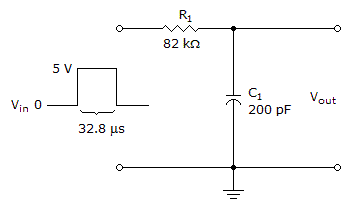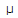# Electronics - Time Response of Reactive Circuits - Discussion

### Discussion :: Time Response of Reactive Circuits - General Questions (Q.No.11)

11.How long will it take the capacitor in the given circuit to discharge?

 [A]. 16.4s [B]. 32.8s [C]. 65.6s [D]. 82s

Explanation:

No answer description available for this question.

 Phaneendra said: (Feb 14, 2011) Solution: Ton+Toff=T f=(1/RC)=6090... 1/f=Toff Toff*5=82microsec.

 Vasu said: (Oct 14, 2011) I dint get last two steps. Y are you multiplying toff*5 ? Whats the correct formula ? anyone please explain ?

 Priya said: (Nov 22, 2011) T=R*C T=82K*200PF=0.0000164 The capacitor will take 5 time constant for fully discharge. So, 0.0000164*5=0.000082 Sec is equal to 82 µs.

 Maneet said: (Oct 5, 2013) How get time constant = 5?

 Saheed said: (Dec 4, 2014) 5 is the Toff period which will be obtained by .0000164/32.8 = 5. 5 times the RC constant = Charging time.